# T3 - page 8216629

...

One more for the T3 collection

Simpler (code) and some additions

• T3Original = true - calculate T3 the original Tim Tilson way
• T3Original = false - calculate T3 Fulks/Matulich modified way
Files:
t3_basic.mq4  4 kb3203

that's the way!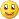thank you, Mladen__________________

//| T3 basic.mq4 |

//| mladen |

//| original T3 developed by Tim Tilson (TASC January 1998) |

//| period lag modification by Bob Fulks and Alex Matulich 4/2003 |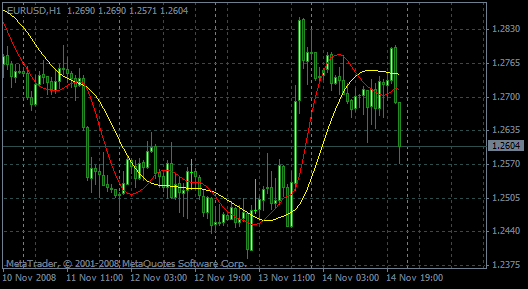pict: org-yellow

T3Period = 14;

T3Price = PRICE_CLOSE;

T3Hot = 0.7;

T3Original = true; /false

Files:
t3_bas_org.gif  13 kb1243

One more for the T3 collection

Simpler (code) and some additions...

Thank you mladen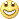nice...will use this in my charts.

Xard7775921

fxbs:
that's the way!thank you, Mladen__________________

//| T3 basic.mq4 |

//| mladen |

//| original T3 developed by Tim Tilson (TASC January 1998) |

//| period lag modification by Bob Fulks and Alex Matulich 4/2003 |pict: org-yellow

T3Period = 14;

T3Price = PRICE_CLOSE;

T3Hot = 0.7;

T3Original = true; /false
mladen:
One more for the T3 collection

Simpler (code) and some additions

Parameters :

• T3Original = true - calculate T3 the original Tim Tilson way
• T3Original = false - calculate T3 Fulks/Matulich modified way
fxbs:
T3_Taotra_NK.mq4 (7.8 KB) -'bands'

T3.new1,2 - T3

Multiple Null Bar Re-Count in Some Indicators - MQL4 Articles

The original Formula for MS 6.5: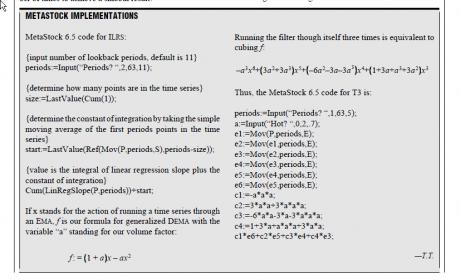METASTOCK IMPLEMENTATIONS

MetaStock 6.5 code for ILRS:

{input number of lookback periods, default is 11}

periods:=Input(“Periods? “,2,63,11);

{determine how many points are in the time series}

size:=LastValue(Cum(1));

{determine the constant of integration by taking the simple

moving average of the first periods points in the time

series}

start:=LastValue(Ref(Mov(P,periods,S),periods-size));

{value is the integral of linear regression slope plus the

constant of integration}

Cum(LinRegSlope(P,periods))+start;

If x stands for the action of running a time series through

an EMA, f is our formula for generalized DEMA with the

variable “a” standing for our volume factor:

f: = 1 + a x – ax2

FIGURE 1: HEWLETT-PACKARD. The EPMA(15), IE/2(15) and ILRS(15) are red,

blue and green, respectively in MetaStock. Note how the EPMA hugs the data more

closely than a simple or exponential moving average of the same length. The price

we pay for this is that it is much noisier than ILRS, and it also overshoots the data

when linear trends are present.

Running the filter though itself three times is equivalent to

cubing f:

–a3x6+ 3a2+3a3 x5+ –6a2–3a–3a3 x4+ 1+3a+a3+3a2 x3

Thus, the MetaStock 6.5 code for T3 is:

periods:=Input(“Periods? “,1,63,5);

a:=Input(“Hot? “,0,2,.7);

e1:=Mov(P,periods,E);

e2:=Mov(e1,periods,E);

e3:=Mov(e2,periods,E);

e4:=Mov(e3,periods,E);

e5:=Mov(e4,periods,E);

e6:=Mov(e5,periods,E);

c1:=-a*a*a;

c2:=3*a*a+3*a*a*a;

c3:=-6*a*a-3*a-3*a*a*a;

c4:=1+3*a+a*a*a+3*a*a;

c1*e6+c2*e5+c3*e4+c4*e3;

It's a shame that NK made the mistake of just code Fulks/Matulich way without a warning to the rest of us.

So, I'm asking me: How sure we are the rest of the Jurik are fine?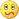Files:3203

Files:
t3series0.mqh  32 kb
t3series.mqh  31 kb
t3series1.mqh  29 kb612

mladen:
One more for the T3 collection

Simpler (code) and some additions

Parameters :

• T3Original = true - calculate T3 the original Tim Tilson way
• T3Original = false - calculate T3 Fulks/Matulich modified way

Thanks Mladen.3203

t3_wpr_cross_multi.mq4 (multipair) Dm_35

Files:98

mladen:
One more for the T3 collection

Simpler (code) and some additions

Parameters :

• T3Original = true - calculate T3 the original Tim Tilson way
• T3Original = false - calculate T3 Fulks/Matulich modified way

Very interesting! And the indicator looks great.

Do you have some documentation on this that can be found online, or that can be easily posted?3203

well. NK worked on digifilters ad Juriks - that was a priority

he couldn't include everything in one time

(and who can?)3203

GlobalTrend-T01en.mq4 (2.7 KB) Tartan

extern int t3_period = 21;

extern double b = 0.7;

extern int kb = 150;

Files: